# Terminology

This list is intended to provide simple explanations for some of the more commonly used dynamic balancing terminology.

### 1．Dynamic Unbalance

Dynamic unbalance is the unbalance that occurs during rotation.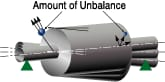### 2．Static Unbalance

Static unbalance is the vector synthesis of lateral unbalance. It is the unbalance that causes a rotor to rotate by gravity when placed on smooth rails.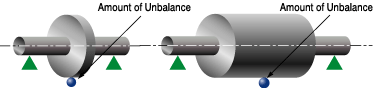### 3．Couple Unbalance

Couple unbalance is the amount of unbalance remaining after correcting static unbalance of rotor. It consists of multiple unbalance vectors of equal magnitude, located laterally apart and oriented 180 degrees in opposite directions.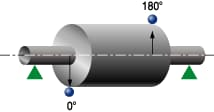### 4．Vertical Dynamic Balancing Machine

Dynamic balancing machine that measures a rotor

### 5．Horizontal Dynamic Balancing Machine

Dynamic balancing machine that measures a rotor

### 6．Soft Type Dynamic Balancing Machine

Dynamic balancing machine using flexible bearing housings for measurements at rotational speeds (frequency) higher than the natural frequency of the unit which uses the bearings.

### 7．Hard Type Dynamic Balancing Machine

Dynamic balancing machine using rigid bearing housings for measurements at rotational speeds lower than the natural frequency of the unit which uses the bearings.

### 8．Dynamic Unbalance

Dynamic unbalance refers to the center of gravity being out of alignment with the center of rotation.

• e : Mass Eccentricity (distance [μm] to center of gravity)
• M : Mass of a Rotor (kg)
• R : Radius of a Rotor (mm)
• m : Mass of a Weight (g)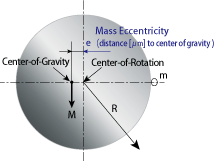### 9．Balancing

Balancing is the process of reducing the amount of unbalance of a rotating object.

• e : Mass Eccentricity (distance [μm] to center of gravity)
• M : Mass of a Rotor (kg)
• R : Radius of a Rotor (mm)
• m : Mass of a Weight (g)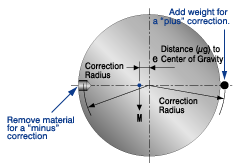### 10．One-Plane Balancing

Process of measuring dynamic unbalance in only one plane in order to measure static unbalance, which is one type of unbalance, or correct the unbalance, especially in thin rotors.### 11．Two-Plane Balancing

Process of measuring dynamic unbalance in two planes to correct one type of dynamic unbalance often found longer rotors.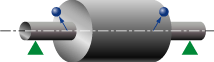### 12．Composite Unbalance

Dynamic unbalance calculated by combining two lateral planes by vector synthesis.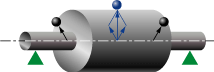### 13．Mass Eccentricity (distance to center of gravity)

Refers to center of rotation being out of alignment. The distance to which it is out of alignment is called the distance to the center of gravity.